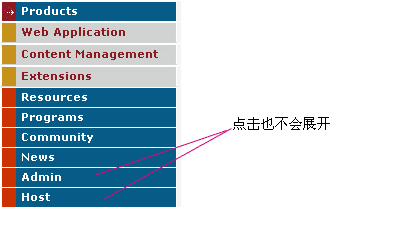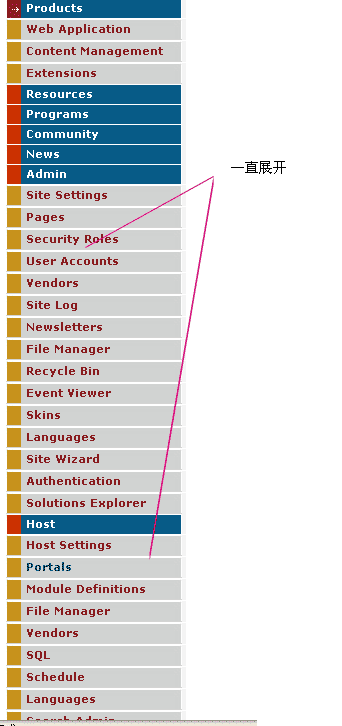+关注继续查看• 找到同名的目录，这里时Vertial1
• 打开vertical1.xsl
• 找到这几行<!--   LEVEL1    --><xsl:if test="Level =1"><xsl:choose ><xsl:when test="( ( ((IsParent ='True') or (Sibling_of_Current_Tab='True')  or DirectChild_of_Current_Tab='True') ) or ($ExpandAll='True' and$Deep>=1)) and (IsCurrent='False')"><tr><td></td></tr></xsl:when>

• 在判断条件test="( ( ((IsParent ='True') or (Sibling_of_Current_Tab='True')  or DirectChild_of_Current_Tab='True') ) or ($ExpandAll='True' and$Deep>=1)) and (IsCurrent='False')"的最后加入一个or (IsAdminTab='True'”（不包括双引号）
• 修改完如下：<!--   LEVEL1    --><xsl:if test="Level =1"><xsl:choose ><xsl:when test="( ( ((IsParent ='True') or (Sibling_of_Current_Tab='True')  or DirectChild_of_Current_Tab='True') ) or ($ExpandAll='True' and$Deep>=1)) and (IsCurrent='False') or (IsAdminTab='True')"><tr><td></td></tr></xsl:when>XCode出现Could not locate device support files的解决办法
XCode出现Could not locate device support files的解决办法
6 0Linux内存管理－高端内存(二)

1044 01308 06 0IntelliJ IDEA常见问题解决办法汇总
IntelliJ IDEA常见问题解决办法汇总
762 0Eclipse遇到Initializing Java Tooling解决办法
1739 02603 01216 03333 0Linux内存管理－高端内存(一)

772 0
412

0

《Nacos架构&原理》

《看见新力量：二》电子书